Question

# Sketch a solid 2 dimensional shape with a center of mass that falls outside the shape

Sketch a solid 2 dimensional shape with a center of mass that falls outside the shape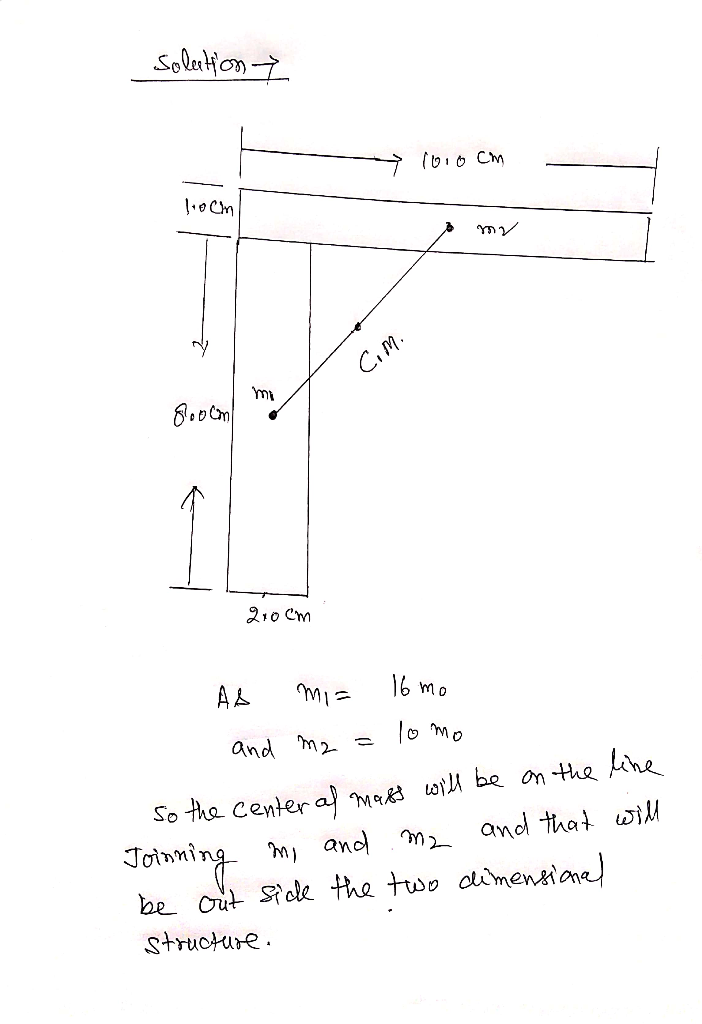#### Earn Coins

Coins can be redeemed for fabulous gifts.

Similar Homework Help Questions
• ### Find the center of mass of a solid of constant density that is bounded by x=y^2 and the planes x=...

Find the center of mass of a solid of constant density that is bounded by x=y^2 and the planes x=z,z= 0 and x= 1. Sketch the solid.

• ### (ii) Density of solid substance with regular shape Cube Shape of the solid substance 122.52 Mass...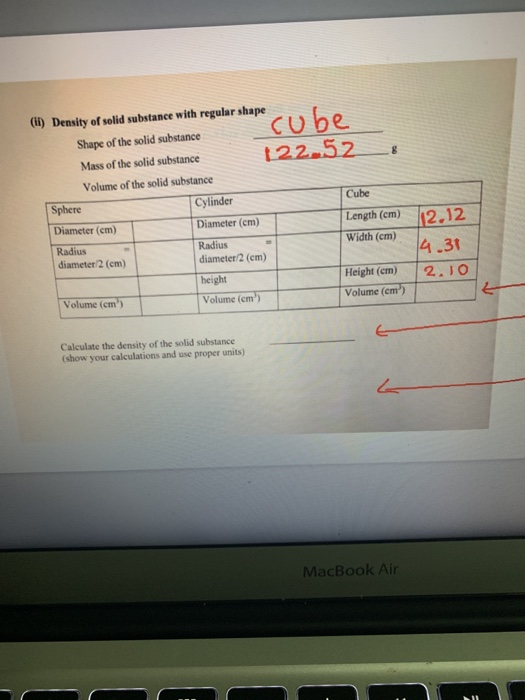(ii) Density of solid substance with regular shape Cube Shape of the solid substance 122.52 Mass of the solid substance Volume of the solid substance Cylinder Cube Length (cm) Diameter (cm) Diameter (cm) Width (cm) Radius Radius diameter 2 (cm) diameter 2 (cm) height Height (cm) Volume (cm) Volume (cm') Volume (cm') Sphere 112.12 la 31 2.10 Calculate the density of the solid substance (show your calculations and use proper units) MacBook Air cube (ii) Density of solid substance with...

• ### 6. Consider a two dimensional mass distribution in the shape of a thin pizza slice: The mass is uniformly distributed a...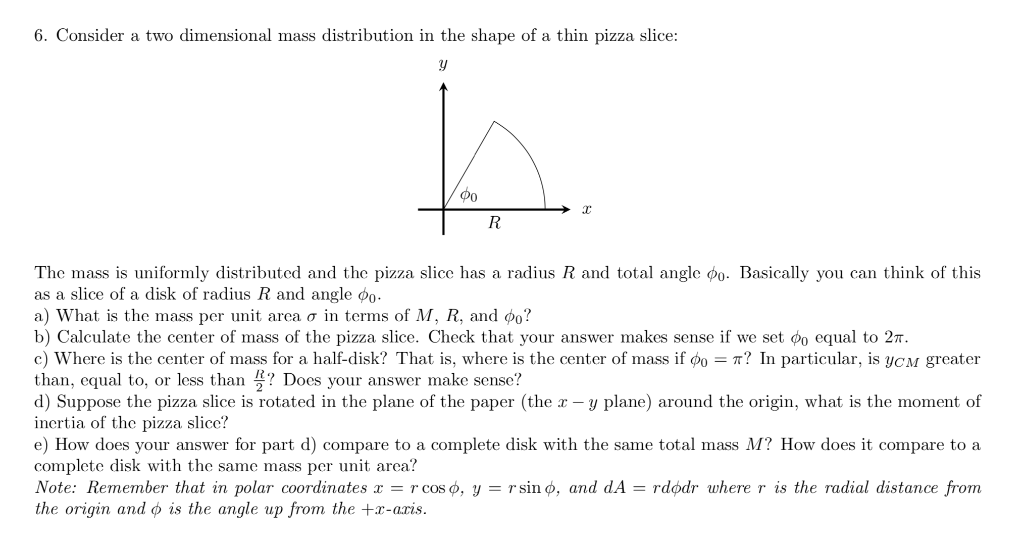6. Consider a two dimensional mass distribution in the shape of a thin pizza slice: The mass is uniformly distributed and the pizza slice has a radius R and total angle o. Basically you can think of this as a slice of a disk of radius R and angle φ。. a) What is the mass per unit area σ in terms of M, R, and φ? b) Calculate the center of mass of the pizza slice. Check that your answer...

• ### 5. Find the center of mass of a solid of constant density & located in the...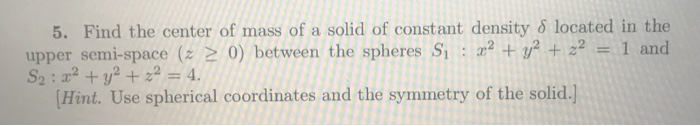5. Find the center of mass of a solid of constant density & located in the upper semi-space (z 2 0) between the spheres S: r + y2 + 22 = 1 and S2: a2+ z2 = 4. Hint. Use spherical coordinates and the symmetry of the solid. 5. Find the center of mass of a solid of constant density & located in the upper semi-space (z 2 0) between the spheres S: r + y2 + 22 = 1...

• ### 1. Construct a model of a methane (CHA) molecule, Sketch the three-dimensional sin ccule. Sketch the three-dimensi...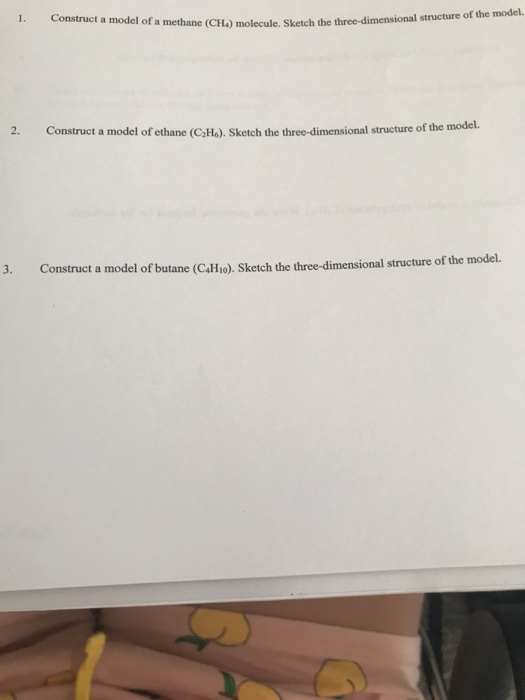1. Construct a model of a methane (CHA) molecule, Sketch the three-dimensional sin ccule. Sketch the three-dimensional structure of the model. 2. Construct a model of ethane (CH), Sketch the three-dimensional structure of the model. 3. Construct a model of butane (CH), Sketch the three-dimensional structure of the model. 3. Molecular Shapes Take a molecular model kit from the cart. Please read the cover of the kit and know what color represent which element. Black is used for carbon white...

• ### 3. A particle of mass m initially at rest falls to the center of the field...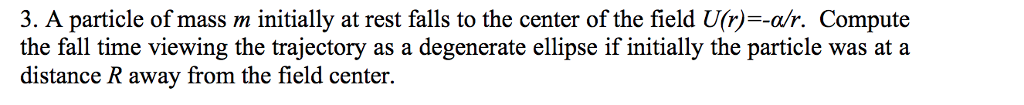3. A particle of mass m initially at rest falls to the center of the field U(r)--a/r. Compute the fall time viewing the trajectory as a degenerate ellipse if initially the particle was at a distance R away from the field center.

• ### 2. Find the center of mass of the solid inside the sphere of radius a >...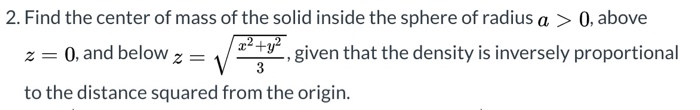2. Find the center of mass of the solid inside the sphere of radius a > 0, above z= 0, and below x2 + y2 given that the density is inversely proportional 3 to the distance squared from the origin.

• ### Find the total mass M and the center of mass of the solid with mass density σ(x, y, z)-kxy3(9-2) ...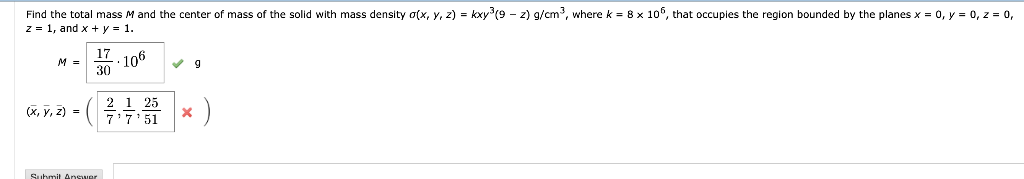Find the total mass M and the center of mass of the solid with mass density σ(x, y, z)-kxy3(9-2) g/cm3, where k z-1, and x + y-1. 2 8 x 106, that occupies the region bounded by the planes x = 0, y 0,2-0. 17 6 30 2 1 25 77 51 (x, y, z) Find the total mass M and the center of mass of the solid with mass density σ(x, y, z)-kxy3(9-2) g/cm3, where k z-1, and x...

• ### Find the mass and center of mass of the solid E with the given density function...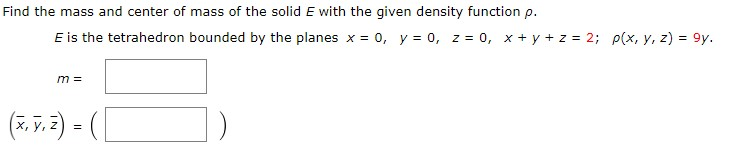Find the mass and center of mass of the solid E with the given density function p. E is the tetrahedron bounded by the planes x = 0, y = 0, z = 0, x + y + z = 2; p(x, y, z) = 9y. m = (7,5,7) = ( [

• ### Question 8.6. The solid inside the sphere x? + y2 + 2? 3 4 and outside...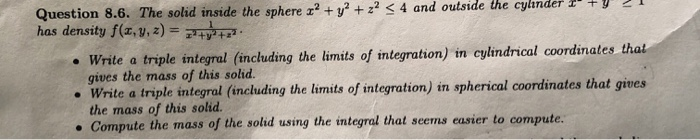Question 8.6. The solid inside the sphere x? + y2 + 2? 3 4 and outside the cylinder I TY has density f(x, y, z) = typ • Write a triple integral (including the limits of integration) in cylindrical coordinates that gives the mass of this solid. • Write a triple integral (including the limits of integration) in spherical coordinates that gives the mass of this solid • Compute the mass of the solid using the integral that seems easier...